# Cohomology of complex projective space

This article describes the value (and the process used to compute it) of some homotopy invariant(s) for a topological space or family of topological spaces. The invariant is cohomology and the topological space/family is complex projective space
Get more specific information about complex projective space | Get more computations of cohomology

## Statement

### With coefficients in the integers$H^p(\mathbb{P}^n(\mathbb{C});\mathbb{Z}) = \left\lbrace\begin{array}{rl} \Z, & \qquad p \ \operatorname{even}, 0 \le p \le 2n\\ 0, & \qquad \operatorname{otherwise}\\\end{array}\right.$

### With coefficients in an abelian group or module

For coefficients in an abelian group$M$, the homology groups are:$H^p(\mathbb{P}^n(\mathbb{C});M) = \left\lbrace\begin{array}{rl} M, & \qquad p \ \operatorname{even}, 0 \le p \le 2n\\ 0, & \qquad \operatorname{otherwise}\\\end{array}\right.$

## Cohomology ring structure

### With coefficients in the integers

The cohomology ring with coefficients in the integers is given as: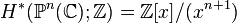$H^*(\mathbb{P}^n(\mathbb{C});\mathbb{Z}) = \mathbb{Z}[x]/(x^{n + 1})$

where the following are true:

• The base ring of coefficients is identified with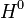$H^0$.
•$x$ (or rather, its image mod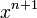$x^{n+1}$) is identified additive generator for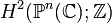$H^2(\mathbb{P}^n(\mathbb{C});\mathbb{Z})$. Note that we could pick either of the two additive generators, since the group is isomorphic to$\mathbb{Z}$.
• Each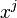$x^j$ is identified with an additive generator for$H^{2j}(\mathbb{P}^n(\mathbb{C});\mathbb{Z})$. In particular,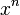$x^n$ is identified with a generator for the top cohomology, or a fundamental class in cohomology.

• The only ring automorphisms of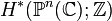$H^*(\mathbb{P}^n(\mathbb{C});\mathbb{Z})$ arising from self-homeomorphisms of the complex projective space are the identity map and the automorphism that acts as the negation map on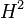$H^2$ and induces corresponding multiplication by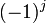$(-1)^j$ maps on each$H^{2j}, 0 \le j \le n$.
• In particular, this means that if$n$ is even, then the top cohomology class is rigid under automorphisms, i.e., there is no automorphism that acts as the negation map on the top cohomology.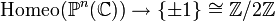$\operatorname{Homeo}(\mathbb{P}^n(\mathbb{C})) \to \{ \pm 1 \} \cong \mathbb{Z}/2\mathbb{Z}$
which sends a homeomorphism to 1 if it acts as the identity on$H^2$ and -1 otherwise.
• More generally, for any continuous self-map of$\mathbb{P}^n(\mathbb{C})$, we can find an integer$m$ such that this map induces multiplication by$m$ on$H^2$. Consequently, it induces multiplication by$m^j$ maps on each$H^{2j}, 0 \le j \le n$.